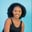Related Tags

python3
pandas

# How to use the Series.ge() function in pandasMaria Elijah

## The Series.ge() function

The pandas Series.ge() function compares two series element-wise, and returns True if the series a is greater than or equal to series b. Otherwise, False is returned.

### Syntax

Series.ge(other, level=None, fill_value=None, axis=0)


### Parameters:

• other: This represents the other series to be compared.

• level (optional): The default value is None. It represents the level to be broadcasted (in the case of multilevel).

• fill_value: This represents the value to replace the missing values in the series. If the element in both corresponding series indexes is NaN, the result of filling at that specific index will be None. The default value is None.

• axis: This indicates the axis to perform the operation on. Value 0 indicates rows and 1 indicates columns. The default is 0.

### Example

The following code will demonstrate how to use the Series.ge() function in pandas:

import pandas as pd
import numpy as np

# create Series
a = pd.Series([17, np.nan, 9, np.nan, 23, 7, np.nan])

b = pd.Series([np.nan, 10, 28, 15, 23, 40, np.nan])

# value to fill nan
replace_nan = 1

# finds  greater or equal element  between the two Series
#  using Series.ge()
print(a.ge(b, fill_value=replace_nan))

### Explanation

In the code above:

• Line 1–2: We import the pandas and NumPy libraries.

• Lines 5–7: We create two series, a and b.

• Lines 10: Then, we create a variable, replace_nan, and assign a value to it. This replaces the NaN values in the series.

• Line 13: We find the greater or equal to element in Series a and b using the ge() function.

Note: If the element in both corresponding series indexes is NaN, the default value for fill_value will be used.

RELATED TAGS

python3
pandas

CONTRIBUTORMaria Elijah
RELATED COURSES

View all Courses

Keep Exploring

Learn in-demand tech skills in half the time